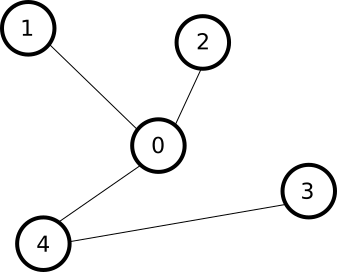시간 제한 메모리 제한 제출 정답 맞은 사람 정답 비율
2 초 1024 MB 10 4 4 40.000%

## 문제

In a far away kingdom, there are $N$ cities numbered between $0$ and $N - 1$. The cities are connected by $N - 1$ two-way roads. Each road has the same length, and connects exactly two cities, such that there is a unique path between any pair of cities.

For any two cities $A$ and $B$, denote by $L(A, B)$ the number of roads of this unique path between cities $A$ and $B$. Given an integer $K$, for how many pairs of cities $A, B$ is $L(A, B) = K$?

## 예제

Let the kingdom have $N = 5$ cities, connected by roads as in the figure below:The following 4 pairs have a single road between them: $(0, 1), (0, 2), (0, 4), (3, 4)$.

The following 4 pairs have two roads between them: $(0, 3), (1, 2), (1, 4), (2, 4)$.

The following 2 pairs have three roads between them: $(1, 3), (2, 3)$.

## 구현

This means that if for $K = 1, 2, 3$, the answers would be $4, 4, 2$ respectively. Your task is to compute how many pairs of cities have exactly $K$ roads between them. You will implement the function paths(N, K, F, T).

• paths(N, K, F, T) - this function will be called exactly once by the judge.
• N: the number of cities in the kingdom.
• K: the number of roads between pairs of cities we are interested in.
• F: an array with $N - 1$ elements. F[i] ($0 \le i < N$) contains one of the cities that the $i$:th road connects.
• T: an array with $N - 1$ elements. T[i] ($0 \le i < N$) contains the other city that the $i$:th road connects.
• It is always possible to travel between any pair of cities using the roads.
• The function should return the number of pairs of cities that has exactly $K$ roads between them.

A code skeleton containing the function to be implemented, together with a sample grader, can be found at attachments.zip.

## 입력

The sample judge reads input in the following format:

• line $1$: N K
• line $2$: F F .. F[N - 2]
• line $3$: T T .. T[N - 2]

## 출력

The sample judge will write a single line with the return value of paths(N, K, F, T).

## 제한

• $1 \le K \le N \le 100\,000$

## 예제 입력 1

5 2
0 0 0 3
1 2 4 4


## 예제 출력 1

4


## 제출할 수 있는 언어

C++17, Java 8, C++14, Java 8 (OpenJDK), Java 11, C++20, Java 15, C++14 (Clang), C++17 (Clang), C++20 (Clang)

## 채점 및 기타 정보

• 예제는 채점하지 않는다.